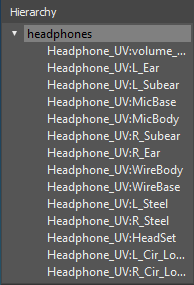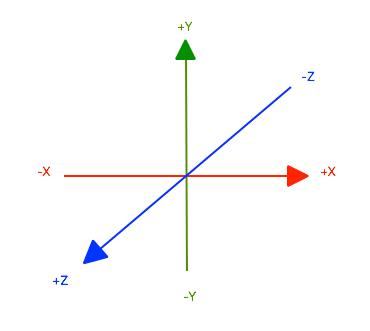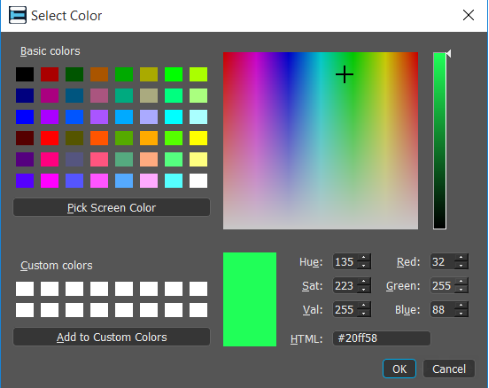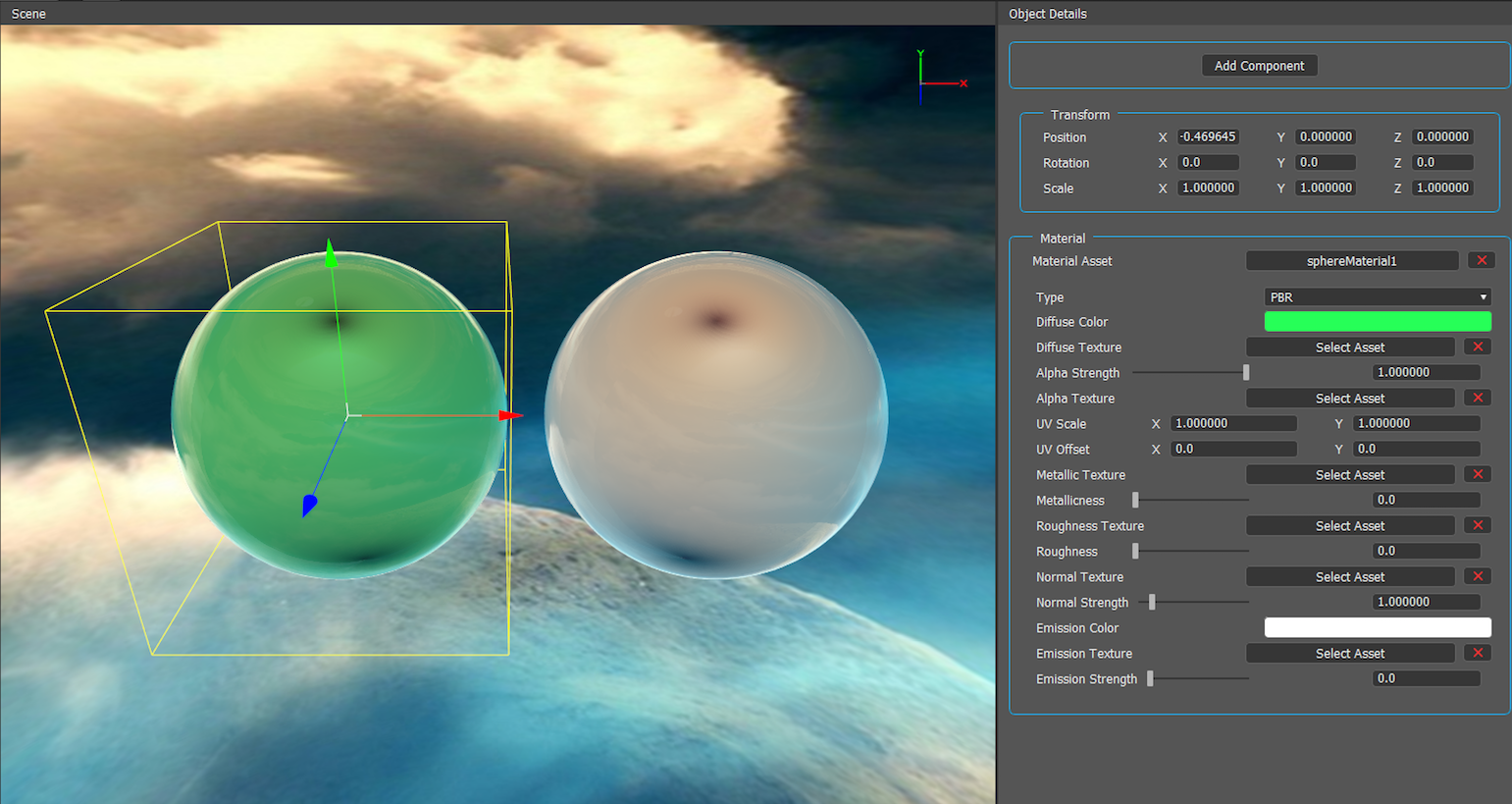# Classes & Globals

Javascript API Classes and Global Properties & Functions

# ObjectDescription

``````Every object in the scene hierarchy is essentially an Object type.
``````

Properties

### name: String

``````Represents the name of this object.
``````

### enabled: Boolean

``````Whether the object is visible or not.
``````

### id: Number

``````Returns the instance id of this object.
``````

### animation: Animation

``````Returns the animation component, or null if there is no such component on this object
``````

### audio: Audio

``````Returns the audio component, or null if there is no such component on this object
``````

### light: Light

``````Returns the light component, or null if there is no such component on this object
``````

### mesh: Mesh

``````Returns the mesh component, or null if there is no such component on this object
``````

### button: Button

``````Returns the button component, or null if there is no such component on this object
``````

### transform: Transform

``````Returns the transform component, or null if there is no such component on this object
``````

# Transform

Description

``````A Transform component defines an object's position,
rotation and scale in global and local space.
``````

Properties

### object: Object

``````The object this transform component is attached to.
``````

### position: Vector3

``````The transform's position in world space.
``````
``````// change the global position of an object to the center of the scene.
object.transform.position = new Vector3(0, 0, 0);

// set only the Y property of the vector
// causing the object to be positioned at (0, 1, 0) in global space
object.transform.position.y = 1;
``````

### localPosition: Vector3

``````The transform's position, relative to its parent.
``````
``````// change the global position of an object to the center of the parent object.
object.transform.localPosition = new Vector3(0, 0, 0);
``````

### localScale: Vector3

``````The transform's scale, relative to its parent.
``````
``````// Set the scale of an object to 2 units on each axis, relative to its parent object.
object.transform.localScale = new Vector3(2, 2, 2);
``````

### rotation: Vector3

``````The transform's rotation represented by Euler angles, in global space.
``````
``````// Set the global rotation of an object to 10° on the Y axis
object.transform.rotation.y = 10;
``````

### localRotation: Vector3

``````The transform's rotation represented by Euler angles, relative to its parent.
``````
``````// Set the local rotation of an object to 180° (opposite direction) on the Y axis
object.transform.localRotation.y = 180;
``````

### childCount: Number

``````The amount of objects that are parented by this transform.
``````
``````// recursively iterate on all children, to count the total amount of objects that are under this object in the hierarchy.
var sum = 0;
function countAllChildren(obj) {
sum += obj.transform.childCount;
for(var i = 0; i < obj.transform.childCount; i++) {
countAllChildren(obj.transform.getChildByIndex(i));
}
}
countAllChildren(object);

Log.printMessage("Total child count: " + sum);
``````

### up: Vector3

``````The transform’s up direction.
``````

### right: Vector3

``````The transform’s right direction.
``````

### forward: Vector3

``````The transform’s forward direction.
``````

### parent : Object

``````The current parent of this transform.
``````
``````var myParent = findObjectByName("other object");
function start() {
object.transform.parent = myParent;
}
``````

Functions

### rotate(degrees: Number, axis: Vector3, space: Space = Space.world) : void

``````Rotates the transform by the specified degrees around the specified axis relative to the specified space.
``````
``````function update(deltaTime) {
// Rotate the object 5° on the Y axis per second
object.transform.rotate(5 * deltaTime, Vector3.up);
}
``````

### lookAt(position: Vector3): void

``````Rotates the transform so that its forward direction
points towards the specified position.
``````
``````function update(deltaTime) {
// Say cheese!
object.transform.lookAt(Camera.position);
}
``````
``````//A good example for staying aligned to a surface in SLAM experiences while facing the camera.
function update(deltaTime) {
var cameraZAndXOnly = new Vector3(Camera.position.x, object.transform.position.y, Camera.position.z);
object.transform.lookAt(cameraZAndXOnly);
}
``````

### translate(direction: Vector3, space: Space = Space.world): void

``````Translates the transform in the specified direction, relative to the specified space.
``````
``````const speed = 5;
function update(deltaTime) {
var direction = Vector3.multiply(Vector3.up, speed * deltaTime);

// Translates the object 5 units up per second in global space.
object.transform.translate(direction);
}
``````

### getChildByName(name: String, searchInChildren: Boolean): Object

``````Returns a child transform by the specified name, null if no child was found.
searchInChildren will also search recursively in all children for the specified name.
``````

### getChildByIndex(index: Number): Object

``````Returns a child transform by the specified index, or null if no child exists at that index.
``````

``````Adds the specified childTransform as a child of this transform.
This will remove childTransform from its current parent should it have one.
``````
``````var otherTransform = findObjectByName("other object").transform;
function start() {
}
``````

### removeChild(childTransform: Transform): void

``````Removes the specified childTransform from the Transform’s child list.
You should save a reference for this Transform for accessing it afterwards.
``````
``````// Detach the first child of this transform
object.transform.removeChild(object.transform.getChildByIndex(0));
``````

# Vector3

Description

``````A datatype that represents a 3D vector.
This class also contains static functions for vector manipulation.
``````

The world coordinates used by the WakingApp AR Studio 3D engine are identical to those imposed by OpenGL.Properties

### x : Number

``````The X component of this vector.
``````

### y : Number

``````The Y component of this vector.
``````

### z : Number

``````The Z component of this vector.
``````

### normalized: Vector3

``````A Vector3 which is a normalized (unit vector) representation of this vector.
``````

### length: Number

``````The Length of this vector.
``````

Functions

### normalize(): void

``````Normalize this vector, making its magnitude (length) 1.
``````
``````var posA = new Vector3(1, 1, 1);
var posB = new Vector3(2, 2, 2);

// calculate the vector3 from posA to posB.
var vec = Vector3.subtract(posB, posA);

Log.printMessage("before: " + vec.length);
vec.normalize();
Log.printMessage("after: " + vec.length);
``````

``````Add vec to this vector.
``````

### subtract(vec: Vector3): void

``````Subtract vec from this vector.
``````

### multiply(vec: Vector3): void

``````Multiply this vector by vec.
``````

### multiply(scalar: Number): void

``````Multiply this vector by a scalar.
``````

### divide(scalar: Number): void

``````Divide this vector by a scalar.
``````

Static Properties

### up: Vector3

``````Shorthand for 'new Vector3(0, 1, 0)'.
``````

### right: Vector3

``````Shorthand for 'new Vector3(1, 0, 0)'.
``````

### forward : Vector3

``````Shorthand for 'new Vector3(0, 0, 1)'.
``````

Static Functions

### distance(vec1: Vector3, vec2: Vector3): Number

``````Returns the distance between two vectors.
``````

### dot(vec1: Vector3, vec2: Vector3): Number

``````Returns a dot product of vec1 & vec2.
For normalized vectors dot will return a value
ranging from **-1**(opposite direction) to **1**(same direction).
``````
``````var otherTransform = findObjectByName("other object").transform;

function start() {

var posA = object.transform.position;
var posB = otherTransform.position;
var direction = Vector3.subtract(posB, posA).normalized;

// dot is a number ranging from -1 to 1 since both vectors are normalized.
// dot equals 0 when both transforms are in the exact same position.
// dot is greater than 0 when the other transform is ahead of this transform.
// dot is lower than 0 when the other transform is behind this transform.
var dot = Vector3.dot(object.transform.forward, direction);
Log.printMessage("Result: " + dot);
}
``````

### cross(vec1: Vector3, vec2: Vector3): Vector3

``````Returns a cross product of vec1 & vec2.
``````

### 📘

https://en.wikipedia.org/wiki/Cross_product

``````var vec = new Vector3(1, 1, 1);
var vec2 = new Vector3(2, 2, 2);

function start() {
var cross = Vector3.cross(vec, vec2);
if(cross.length < Number.EPSILON) {
Log.printMessage("Vectors are parallel");
}
}
``````

### lerp(from: Vector3, to: Vector3, t: Number): Vector3

``````Returns a new Vector3 representing the linear interpolation between
vec1 and vec2, by t which is a  value ranging from 0 to 1 for interpolation, and outside the bounds of 0 to 1 for extrapolation
``````
``````const startPosition = new Vector3(1, 1, 1);
const endPosition = new Vector3(2, 2, 2);
const speed = 2;
var t = 0;

function update(deltaTime) {
t += deltaTime;

// move this object from startPosition towards endPosition, the animation time will be (1 / speed) seconds - which in this case is 0.5 seconds.
object.transform.position = Vector3.lerp(startPosition, endPosition, t * speed);

}
``````

### add(vec1: Vector3, vec2: Vector3): Vector3

``````Returns a new Vector3 which is the result of adding vec1 and vec2.
``````

### subtract(vec1: Vector3, vec2: Vector3): Vector3

``````Returns a new Vector3 which is the result of subtracting vec1 by vec2.
``````

### multiply(vec: Vector3, scaler: Number): Vector3

``````Returns a new Vector3 which is the result of multiplying vec1 by vec2 / vec by scaler, respectively.
``````

### divide(vec: Vector3, scaler: Number): Vector3

``````Returns a new Vector3 which is the result of dividing vec by a scalar.
``````

# Vector2

Description

``````A datatype that represents a 2D vector.
This class also contains static functions for vector manipulation.
``````

Properties

### x : Number

``````The X component of this vector.
``````

### y : Number

``````The Y component of this vector.
``````

### normalized: Vector2

``````A Vector2 which is a normalized (unit vector) representation of this vector.
``````

### length: Number

``````The Length of this vector.
``````

Functions

### normalize(): void

``````Normalize this vector, making its magnitude (length) 1.
``````

``````Add vec to this vector.
``````

### subtract(vec: Vector2): void

``````Subtract vec from this vector.
``````

### multiply(vec: Vector2): void

``````Multiply this vector by vec.
``````

### multiply(scalar: Number): void

``````Multiply this vector by a scalar.
``````

### divide(scalar: Number): void

``````Divide this vector by a scalar.
``````

Static Functions

### distance(vec1: Vector2, vec2: Vector2): Number

``````Returns the distance between two vectors.
``````

### dot(vec1: Vector2, vec2: Vector2): Number

``````Returns a dot product of vec1 & vec2.
For normalized vectors dot will return a value
ranging from **-1**(opposite direction) to **1**(same direction).
``````

### lerp(from: Vector2, to: Vector2, t: Number): Vector2

``````Returns a new Vector2 representing the linear interpolation between
vec1 and vec2, by t which is a  value ranging from 0 to 1 for interpolation, and outside the bounds of 0 to 1 for extrapolation
``````

### add(vec1: Vector2, vec2: Vector2): Vector2

``````Returns a new Vector2 which is the result of adding vec1 and vec2.
``````

### subtract(vec1: Vector2, vec2: Vector2): Vector2

``````Returns a new Vector2 which is the result of subtracting vec1 by vec2.
``````

### multiply(vec: Vector2, scaler: Number): Vector2

``````Returns a new Vector2 which is the result of multiplying vec1 by vec2 / vec by scaler, respectively.
``````

### divide(vec: Vector2, scaler: Number): Vector2

``````Returns a new Vector2 which is the result of dividing vec by a scalar.
``````

# Color

Description

``````A datatype that represents a color used by materials in the 3D engine.
This class also contains static functions for color manipulation.
``````Properties

### r : Number

``````Red component of the Color.
``````

### g : Number

``````Green component of the Color.
``````

### b : Number

``````Blue component of the Color.
``````

### a : Number

``````Alpha component of the Color.
``````

Functions

``````Add color to this color
``````

### subtract(color: Color): void

``````Subtract color from this color.
``````

### multiply(scalar: Number): void

``````Multiply this color by color/scalar respectively.
``````

### divide(scalar: Number): void

``````Divide this color by scalar.
``````

Static Functions

### lerp (color1: Color, color2: Color, t: Number): Color

``````Returns a new Color representing the linear interpolation between color1 and color2, by t which is a  value ranging from 0 to 1 for interpolation, and outside the bounds of 0 to 1 for extrapolation
``````

### add(color1: Color, color2: Color): Color

``````Returns a new Color which is the result of adding color1 and color2.
``````

### subtract(color1: Color, color2: Color): Color

``````Returns a new Color which is the result of subtracting color1 by color2.
``````

### multiply(color1: Color, color2: Color): Color

``````Returns a new Color which is the result of multiplying color1 by color2.
``````

### multiply(color: Color, scalar: Number): Color

``````Returns a new Color which is the result of multiplying a color by a scalar.
``````

### divide(color: Color, scalar: Color): Color

``````Returns a new Color which is the result of dividing a color by a scalar.
``````

# Camera

Description

``````A class that holds static (read only) properties for the Camera's transform.
- In WakingApp AR Studio, you have no control over the camera as the AR Tracking mechanism
- is in charge of the camera's position and orientation.
``````

Static Functions

### getIntersectionPointSLAM(): Vector3

``````Returns the intersection point between a ray cast from the center of the screen to the plane generated by the SLAM tracker, useful for placing objects in SLAM type projects.
On the Editor, this function returns the intersection point between the mouse cursor and a plane placed and the center of the world pointing towards (0,1,0).
``````

### getIntersectionRotationSLAM(): Vector3

``````Returns the intersection rotation between a ray cast from the center of the screen to the plane generated by the SLAM tracker, useful for placing objects in SLAM type projects.
``````

Static Properties

### transform: Transform

``````The camera object's transform component (Read only).
``````

### position: Vector3

``````The camera’s position in world space.
``````

Example usage in the lookAt function

### rotation: Vector3

``````The camera’s rotation in world space, represented by Euler angles.
``````

### forward: Vector3

``````The camera’s forward direction.
``````

### right: Vector3

``````The camera’s right direction.
``````

### up: Vector3

``````The camera’s up direction.
``````

# Animation

Description

``````The animation component provides an interface to control animation playback.
Animations are imported if they are included in 3D model files.
``````

Properties

### object: Object

``````The object this animation component is attached to.
``````

### loop: Boolean

``````Determines whether the animation should loop on end, or not.
``````

### playSpeed: Number

``````The current animation playing speed. Clamped between 0 and 2.
``````

### time: Number

``````The current time in the AnimationClip.
Runs from 0 to the ‘length’ property of the AnimationClip.
``````

### clipCount: Number

``````The number of animation-clips stored in the animation.
``````

### clip : AnimationClip

``````The currently set animationClip.
``````
``````function start() {
if(object.animation.clip.name != "idle") {
object.animation.clip = object.animation.getClipByName("idle");
}

object.animation.play();
}
``````

Functions

### play(): void

``````Starts playing the animation.
``````

### pause(): void

``````Pauses the animation. Calling 'play()' will pick it from where it was paused.
``````

### stop(): void

``````Stops the animation, resetting it to its first frame.
``````

### isPlaying(): Boolean

``````Whether the animation is currently playing or not.
``````

### getClipByName(name: String): AnimationClip

``````Returns an AnimationClip with the specified name or null if no clip is found.
``````

### getClipByIndex(index: Number): AnimationClip

``````Returns an AnimationClip by the specified index.
``````

# AnimationClip

Description

``````An AnimationClip represents a set of frames (clip) imported from the 3D model file
``````

Properties

### length: Number

``````Time duration in seconds for this animation clip.
``````

### name: String

``````Returns the clip’s name.
``````

# Audio

Description

``````An audio component provides interface for controlling and manipulating sound.
``````

Properties

``````The object this audio component is attached to.
``````

### loop: Boolean

``````Determines whether the audio should loop on end, or not.
``````

### volume: Number

``````Determines the volume of the audio clip. Clamped between 0 and 1.
``````

### pitch: Number

``````Determines the pitch of the audio clip.
``````

### clip: AudioClip

``````Holds the audio clip property.
``````

Functions

### play(): void

``````Starts audio playback
``````

### pause(): void

``````Pauses the audio playback. Playing it again will pick it from where it was paused.
``````

### stop(): void

``````Stops the audio clip, resetting it to the beginning.
``````

### isPlaying(): Boolean

``````Whether the audio is currently playing or not.
``````

# Light

Description

``````Lights components are used to provide additional lighting (beyond the reflection map) to the PBR material.
``````

Properties

### object: Object

``````The object this lightco mponent is attached to.
``````

### color: Color

``````The color of the light.
``````

### intensity: Number

``````The intensity of the light.
``````

``````For point lights - affects the radius in which light is emitted.
``````

### type: LightType

``````The light's type - Directional, Point or Spot
``````

# Button

Description

``````The button component enables adding intractable buttons on the project's UI layer.
``````

Properties

### object: Object

``````The object this button component is attached to.
``````

### onPressed: Function

``````A callback which gets called whenever the button is pressed.
``````
``````void myFunction()
{
Log.printMessage("Button was pressed!");
}

void start()
{
object.button.onPressed = myFunction;
}
``````

### anchor: Anchor

``````The button's anchor point.
``````

### pivot: Pivot

``````The button's pivot point.
``````

### visible: Boolean

``````Determines whether the button should be rendered.
``````

### text: String

``````Holds the text displayed on top of the button.
``````

### position: Vector2

``````Holds the button position relative to its anchor.
``````

### size: Vector2

``````Holds the button size relative to the project's UI-preview resolution.
``````

### color: Color

``````Holds the button color property.
``````

### texture: Texture

``````Holds the button texture property.
``````

### textColor: Color

``````Holds the button text color property.
``````

### pressedColor: Color

``````Holds the button pressed color property.
``````

### pressedTexture: Texture

``````Holds the button pressed-texture property.
``````

### borderColor: Color

``````Holds the button border color property.
``````

# Label

Description

``````The label component enables adding 2d text labels on the project's UI layer.
``````

Properties

### object: Object

``````The object this label component is attached to.
``````

### anchor: Anchor

``````The label's anchor point.
``````

### pivot: Pivot

``````The label's pivot point.
``````

### visible: Boolean

``````Determines whether the label should be rendered.
``````

### text: String

``````Holds the text displayed on top of the label.
``````

### position: Vector2

``````Holds the label position relative to its anchor.
``````

### size: Vector2

``````Holds the label size relative to the project's UI-preview resolution.
``````

### textColor: Color

``````Holds the label text color property.
``````

# Mesh

Description

``````The mesh component provides an interface for the materials of the mesh,
imported from the 3D Model file.
``````

Properties

### object: Object

``````The object this mesh component is attached to.
``````

### material: Material

``````The material of this mesh if it has one, null otherwise (read only).
The returned material returns as an instance of the correct material type.
``````

# Material

Description

``````Represents any material that can be used by the 3D Engine (PBR, Video, Diffuse).
Provides an interface for manipulating color, controlling video playback etc..
``````

Properties (All Materials)

### name: String;

``````Holds the material name.
``````

### diffuse: Color

``````Holds the material diffuse color property.
``````

### diffuseTexture: Texture

``````Holds the material diffuse texture property.
``````

### type: MaterialType

`````` Holds the material type.
``````

Properties (PBR Material)

### metallic: Number

``````Holds the material metallicness property.
``````

### roughness: Number

``````Holds the material roughness property.
``````

### emissive: Color

``````Holds the material emissive color property.
``````

### normalStrength: Number

``````Holds the material normal strength property.
``````

### roughnessMetallicTexture: Texture

``````Holds the material roughnessMetallic texture property.
``````

### emissionTexture: Texture

``````Holds the material emissiontexture property.
``````

### ambientOclussionTexture: Texture

``````Holds the material ambientOclussion texture property.
``````

Properties (Video Material)

### loop: Boolean

``````Determines whether the video should loop on end, or not.
``````

### volume: Number

``````Determines the volume of the video clip. Clamped between 0 and 1.
``````

### video: Video

``````Holds the material video clip property.
``````

Functions (Video Material)

### playVideo(): void

``````Starts playing the video - on the mesh's surface
``````

### pauseVideo(): void

``````Pauses the video. Playing it again will pick it from where it was paused.
``````

### stopVideo(): void

``````Stops the video, resetting it to the beginning.
``````

### isPlaying(): void

``````Returns whether the video is currently playing or not.
``````

# Global Properties & Functions

Description

``````Global properties and functions are always 'in context' within a script.
``````

## object: Object

``````Holds a reference to the object the script component is attached to.
``````

## findObjectByName(name: string): Object

``````Finds an object in the scene by its name.
The first object found will be returned.
Returns null if no object is found.
``````

## getIntersectionPointSLAM(name: string): Vector3

``````Returns the intersection point between a ray casted from the center of the screen to the plane
generated by the SLAM tracker, useful for placing objects in SLAM type projects.
+ In the Editor, this function returns the intersection point between the mouse cursor and a grid's plane.
``````

## getTrackingStateSLAM(): String

``````Returns the current SLAM tracking state.
+ Possible values : 'none', 'low' and 'normal'.
``````

# Application

Description

``````Class which contains application-wide utility functions.
``````

Static Functions

### openURL(url: String): void

``````Opens the url in a new page with the default browser.
``````

``````
Unloads the currently open scene and loads another scene that is part of the project.
``````

# Input

Description

``````Class which grants access to read user input.
``````

Static Properties

### touchCount: Number

``````The number of currently active screen touches (mobile). On the Editor, the left mouse button will be considered as a single touch.
``````

# Log

Description

``````Use the Log class to print messages/errors to the editor's console.
+ The mobile SDK forwards messages/errors to the device's log.
``````

Static Functions

### printMessage(message: String): void

``````Prints a message to the console (Editor) or the device log (mobile).
``````

### printError(error: String): void

``````Prints an error to the console (Editor) or the device log (mobile).
``````

# AudioClip

Description

``````A class used  to encapsulate .mp3 assets.
``````

# Texture

Description

``````A class used  to encapsulate image-based assets.
``````

# Video

Description

``````A class used  to encapsulate .mp4 video assets.
``````

# WebRequest

Description

``````A class which may be used to invoke web requests.
``````

Properties

### contentType: String

``````The Content-Type header for this webrequest. will be set to application/x-www-form-urlencoded by default.
``````

### onProgress: Function

``````A callback function which gets called whenever a request is in progress. Expected signature : onProgress(WebRequest request, Number bytesRecieved, number bytesTotal)
``````

### onComplete: Function

``````A callback function which gets called whenever a request is complete. Expected signature : onComplete(WebRequest request)
``````

### onFailure: Function

``````A callback function which gets called whenever a request fails. Expected signature : onFailure(WebRequest request, String errorMessage)
``````

Functions

### get(url: String) : void

``````Invokes a GET web request
``````

### post(url: String, postData : object) : void

``````Invokes a POST web request.
``````

### responseAsString() : String

``````Returns the bytes of a completed request as string. Must be called after onComplete has been invoked.
``````

### responseAsTexture() : Texture

``````Returns the bytes of a completed request as a Texture. Must be called after onComplete has been invoked.
``````

### responseAsAudioClip() : AudioClip

``````Returns the bytes of a completed request as an AudioClip. Must be called after onComplete has been invoked.
``````

### responseAsVideo() : Video

``````Returns the bytes of a completed request as an Video. Must be called after onComplete has been invoked.
``````
``````//example WebRequest usage

function onProgressCallback(request, bytesWritten, bytesTotal)
{
Log.printMessage("request progress :  " + bytesWritten + " / " + bytesTotal +" bytes");
}

function onCompleteCallback(request)
{
//create a texture from the request's response bytes and set it to the material's diffuse texture
object.mesh.material.diffuseTexture = request.responseAsTexture();
}

function onFailureCallback(request, errorMessage)
{
Log.printError("request failed with error: " + errorMessage);
}

function start()
{
//example GET request obtaining an image from a .png url
var myRequest = new WebRequest();
myRequest.onProgress = onProgressCallback;
myRequest.onComplete = onCompleteCallback;
myRequest.onFailure = onFailureCallback;
myRequest.get("https://sample-videos.com/img/Sample-png-image-100kb.png");

//example POST request sending login credentials
var myPostRequest = new WebRequest();
myPostRequest.contentType = "application/x-www-form-urlencoded";
}
``````